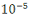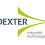# Intrinsic and Extrinsic Semiconductor - Engineering Physics

Semiconductor

A semiconductor is a substance which can be understood by his name that means it is not a pure conductor but can become conductor by applying some conditions otherwise it behaves like an insulator. Now, in this case, condition means by increasing temperature. At 0K most semiconductors would be an insulator, but while we increase the temperature, charge carriers in the semiconductors get the energy to move. To understand the main difference between conductor, semiconductor, and insulator, we have to understand the meaning of the forbidden gap. The forbidden gap is the gap between the highest filled band (valence band) and the first empty band (conduction band). It is relatively small in semiconductors then insulators. In conductor, electric current conducted by negative charge carrier alone but in semiconductors electric current can be conducted by two types of charge carriers, one type of charge carrier is a negative charge (electrons) and another type of charge is positively charge (holes).

Characteristic properties of semiconductors

1. The resistivity of semiconductors decrease sharply with an increase of temperature i.e. it has negative temperature coefficient of resistance.

3. Their electrical conductivity is very much affected by adding a small amount of impurity to it.

4. A junction made by a p-type semiconductor and n-type semiconductor shows rectification property.

5. Some semiconductors are sensitive to light and they produce a photo-voltage.

Intrinsic Semiconductor

Intrinsic means pure semiconductor. Generally on the basis of electrical conductivity semiconductor may be defined as a substance that has resistivity (toΩm) in between conductors and insulators and it decreases with rising in temperature of the semiconductor. Mainly two elements Ge and Si are regarded as basic semiconductors. Semiconductors like germanium and silicon have a crystalline structure. Their crystals have a cubic lattice structure similar to diamond. They are tetravalent, i.e., each has four valence electrons in its outermost shell.

A pure Ge atom has 32 electrons out of which 28 are tightly bound to the nucleus and the remaining 4 revolve in the outermost level, similarly, the Si atom has 14 electrons out of which 10 are tightly bound to the nucleus and the remaining 4 revolve in the outermost level. The valence electrons are held by covalent bonds with the valence electrons of four adjacent germanium atoms, fig. A considerable amount of energy is needed to disturb these bonds. The covalent bonds of pure Ge at 0K behaves as a non-conductor of electricity as there is no free carrier of charge. When we increase the temperature, some covalent bonds are broken due to thermal agitation. Thus some of the electrons become free. These electrons move freely through the crystal lattice in a random manner.

In early solid-state devices like p-n junctions and transistors, Ge has been used but now it has been replaced entirely by Si due to its availability in abundance. At present mostly the complicated integrated circuits (IC) are manufactured from Si.

Extrinsic Semiconductors –

Intrinsic semiconductors have very low conduction capability. To increase the conduction capabilities of semiconductors, we have to add a small amount of suitable impurity. Those impure semiconductors are known as extrinsic semiconductors. The impurity atom is known as dopant, the semiconductor containing impurity is called doping and the process of adding impurity is called doping. The impurity is added either to increase the number of electrons or the number of holes. Since semiconductor generally belongs to the fourth group of the periodic table, So the impurities which are added are elements belonging to the third and the fifth group of the periodic table. They are commonly boron, gallium, indium, and aluminum from the third group which is trivalent and phosphorous, antimony, and arsenic from the fifth group which is pentavalent.

Depending upon the type of impurity added, extrinsic semiconductors are classified into

1. n-type semiconductor

2. p-type semiconductor

1. n-type semiconductor

In n-type semiconductor an extremely small amount of impurity from the fifth group of the periodic table says As or Sb is added to the highly pure Ge or Si semiconductor. Since each atom of impurity has five valence electrons, so one valence electron is left over after the structure of the covalent bond yet it is not completely detached from the impurity atom itself. Some amount of energy is required by this electron to completely detach itself from the attractive force of the positive nucleus of the impurity atom, called ionization energy.

In Ge, the ionization the energy of As, Sb and P atom is approximately 0.001eV and in Si, the ionization energy of impurity like As, Sb and P are 0.045eV, 0.039eV and 0.049eV respectively. It may be noted that by giving away its one valence electron, the donor atom becomes a positively charged ion, but it cannot take part in conduction because it is not free but tied to the crystal lattice.

Consequently, donor impurities contribute conduction electrons to the semiconductor without contributing holes. Hence the concentration of electrons in the conduction band is increased and exceeds the concentration of holes in the valence band.

Thus the Fermi level shifts upwards towards the bottom of the conduction band as shown in fig. The donor level is just below the conduction band. Thus electron density in n-type a semiconductor is very large as compared to the hole density, thus electrons are the majority current carriers and holes are the minority current carriers in this type of semiconductor.

2. p-type semiconductor

In p-type semiconductor extremely small amount of impurity from the third group of the periodic table say Zn or Ga is added to the highly pure Ge or Si semiconductors. Since each atom of impurity has three valence electrons, so there is a vacancy in the covalent bond structure or a hole associated with each accepter atom. Though a hole is nothing but an electron vacancy, it actually behaves like a positively charged particle. The exact situation is shown in fig(a). Since there is a strong tendency of semiconductor crystals to form covalent bonds, hence, a hole attracts one electron from a nearby covalent bond, consequently, a new hole is created at the originally occupied place of an electron. This hole is again filled by another electron from a nearby bond giving rise to another hole and so on. Thus a hole moves freely through the crystal lattice. Since concentration of holes in the valence bond are more than the concentration of electrons in the conduction band, Fermi level shifts nearer the valence band as shown in fig (b). The acceptor level lies immediately above the Fermi level. Conduction is by means of hole movement at the top of the valence band, the acceptor level readily accepting electrons from the valence band.

In p-type semiconductors holes are the majority of current carriers and electrons are minority carriers.

1.